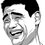# Calculating effective mass when Spring itself is massive in Mechanics problems.

So, this is a post regarding a general result which is of a lot of help if remembered in Mechanics problems. The derivation is of how to calculative effective mass of block and spring attached if spring is massive.

Let's consider the mass of spring to be $m$.

So let's begin,

So let us take a small element of mass $dm$ of the spring, so total energy can be calculated by adding all the length elements' kinetic energy, and requires the following integral:

$E = \int \dfrac{1}{2}u^{2} dm$

Since spring is uniform,

$dm = (\dfrac{dy}{L})m$

$E = \int_{0}^{L} \dfrac{1}{2}u^{2}(\dfrac{dy}{L})m$

The velocity of each mass element of the spring is directly proportional to its length, i.e.

$u = (\dfrac {vy}{L})$

$E = \dfrac{1}{2} \dfrac{m}{L} \int_{0}^{L} (\dfrac{vy}{L})^{2} dy$

$E= \dfrac{1}{2} \dfrac{m}{3} v^{2}$

Comparing to the expected original kinetic energy formula,

$K.E. = \dfrac{1}{2}mv^{2}$

we can conclude that effective mass of spring in this case is $\dfrac{m}{3}$

The EFFECTIVE MASS of the spring in a spring-mass system when using an ideal spring of uniform linear density is $\dfrac{1}{3}$ of the mass of the spring.

And we're done.Note by Ritvik Choudhary
6 years, 5 months ago

This discussion board is a place to discuss our Daily Challenges and the math and science related to those challenges. Explanations are more than just a solution — they should explain the steps and thinking strategies that you used to obtain the solution. Comments should further the discussion of math and science.

When posting on Brilliant:

• Use the emojis to react to an explanation, whether you're congratulating a job well done , or just really confused .
• Ask specific questions about the challenge or the steps in somebody's explanation. Well-posed questions can add a lot to the discussion, but posting "I don't understand!" doesn't help anyone.
• Try to contribute something new to the discussion, whether it is an extension, generalization or other idea related to the challenge.

MarkdownAppears as
*italics* or _italics_ italics
**bold** or __bold__ bold
- bulleted- list
• bulleted
• list
1. numbered2. list
1. numbered
2. list
Note: you must add a full line of space before and after lists for them to show up correctly
paragraph 1paragraph 2

paragraph 1

paragraph 2

[example link](https://brilliant.org)example link
> This is a quote
This is a quote
    # I indented these lines
# 4 spaces, and now they show
# up as a code block.

print "hello world"
# I indented these lines
# 4 spaces, and now they show
# up as a code block.

print "hello world"
MathAppears as
Remember to wrap math in $$ ... $$ or $ ... $ to ensure proper formatting.
2 \times 3 $2 \times 3$
2^{34} $2^{34}$
a_{i-1} $a_{i-1}$
\frac{2}{3} $\frac{2}{3}$
\sqrt{2} $\sqrt{2}$
\sum_{i=1}^3 $\sum_{i=1}^3$
\sin \theta $\sin \theta$
\boxed{123} $\boxed{123}$

Sort by:

how we came to know that velocity of each mass element of the spring is directly proportional to its length????

- 6 years, 5 months ago

It's true that this result is based only on the assumption that velocity of each mass element of the spring is directly proportional to its length, but since above is technique used for solving certain problems in classical mechanics where you can generally take the above assumption as valid.

- 6 years, 5 months ago

Thanks Ritvik

- 6 years, 4 months ago

- 6 years, 4 months ago

This is only True when we assume Liniar relation of velocity of massive spring ! So it is not the genral result !

- 6 years, 5 months ago

how can we assume it..?? can you pls explain how is velocity linearly proportional to distance of point from the fixed end..

- 3 years, 4 months ago

It is only true when the spring is stretching/compressing uniformly,i.e. when the end point of the spring is stretched by x, then the mid-point is stretched by (x/2). So the element of the spring which is at y distance from the origin should stretch by (xy/L) , where L is the length of the spring. So from this we can see that the velocity of that element is (vy/L) where v is the velocity of the end point of the spring. Hope this helps..

- 3 years, 3 months ago